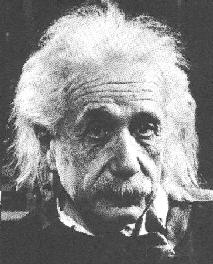#Physics Concepts

Let's have a look at how you can use Newton's Second Law to figure out how fast your rocket will go. First we need to understand

then we'll put them together to find your rocket's speed.

If you are already familiar with these concepts you may want to go on to the Applied Physics page, where I show how to use these equations to find the right motor for your rocket.

You might notice that we make all our measurements here using the metric system. That's because the metric system is designed for all the equations to work without any conversion factors just to fix the funny units. If we measure in English units, feet and inches and pounds, then there are fudge factors all over the place... very confusing.

## Velocity

This is the measure of your speed, defined as the distance covered each second, or each minute, or each hour. In equation form, then, you can find the distance you've covered by multiplying the velocity at which you're travelling by the time you're going at that speed:

Distance = velocity x time

or in short form,

s = v x t

Example:

Toy car rolling at 2 meters per second -
After the car has gone
1 second 2 meters
2 seconds 4 meters
3 seconds 6 meters
4 seconds 8 meters

## Acceleration

But now what if your velocity is changing, like when you're rocket's engine is burning and the rocket is rapidly speeding up? How do you measure how you're speeding up?

The term for this is acceleration, and the measure is to figure out how much faster you're going after each second (or after each minute or hour). If it takes my car 10 seconds to go from 0 to 60 miles per hour, then my acceleration is 6 miles per hour each second - we would say 6 miles per hour per second.

If I measure velocity in meters per second instead of miles per hour, then acceleration is in meters per second per second. It sounds funny but that's how you say it.

So now if I know my acceleration and how long I accelerate, I can find my velocity (speed) by multiplying the acceleration times the time, or in equation form,

Velocity = acceleration x time

or in short form,

v = a x t

Example:

Car accelerating at 5 meters per second per second -
After the car is going
1 second 5 meters per second
2 seconds 10 meters per second
3 seconds 15 meters per second
4 seconds 20 meters per second

Note: 1 meter per second (m/s) equals 2.237 miles per hour.

## Force

Newton's Second Law:

Force = mass x acceleration, or

F = m x a (sometimes written F = ma)

You usually think of force in pounds - in the metric system force is measured in "Newtons", yes, named after Sir Isaac. A Newton is not a lot of force - one pound is 4.45 Newtons.

Example:

Pushing a 0.25 kilogram rocket -
Motor's thrust rocket's acceleration
1 newton 4 meters/sec/sec
2 newtons 8 meters/sec/sec
3 newtons 12 meters/sec/sec
4 newtons 16 meters/sec/sec

## So What?

So now you can find the speed your rocket will go:

If I know the force applied to the rocket, and the mass of the rocket, I can use Newton's second law to find the acceleration.

Once I know my acceleration and how long I accelerate, I can find velocity (v = a x t).

Example:

My rocket weighs 3.5 ounces = 0.1 kg.
Note: 1 kilogram equals 35.27 ounces

If we use a "C5" motor, it will have a thrust of 5 Newtons for 2 seconds, according to its specifications.

That's all we need to know! Putting it all together, we have:

F = 5 newtons

m = 0.1 kg

t = 2 seconds

F = m x a, therefore 5 = 0.1 x a, so a = 50 meters/sec/sec

v = a x t = 50 x 2 = 100 meters/second = 223.7 mph!

...and you won't get a ticket! Some of the faster model rockets will get up to 300 mph and even more, so this speed is actually pretty typical.On to Applied Physics: how to use these equations to find the right motor for your rocket.Back to Rocket Simulation

## Questions

Your questions and comments regarding this page are welcome. You can e-mail Randy Culp (Tripoli #6926) for inquiries, suggestions, new ideas or just to chat.
Updated 24 August 2008